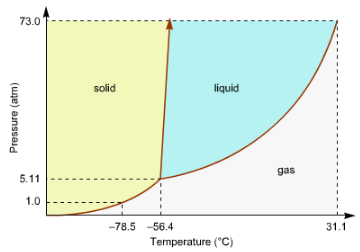# Problem: Consider this phase diagram for carbon dioxide.a. In what phase is CO2 at 72 atm and 0 degree C? b. Starting from the point described above, what phase change would eventually result from a decrease in pressure?

###### FREE Expert Solution
86% (251 ratings)
###### Problem Details

Consider this phase diagram for carbon dioxide.

a. In what phase is CO2 at 72 atm and 0 degree C?

b. Starting from the point described above, what phase change would eventually result from a decrease in pressure?What scientific concept do you need to know in order to solve this problem?

Our tutors have indicated that to solve this problem you will need to apply the Phase Diagram concept. You can view video lessons to learn Phase Diagram. Or if you need more Phase Diagram practice, you can also practice Phase Diagram practice problems.

What is the difficulty of this problem?

Our tutors rated the difficulty ofConsider this phase diagram for carbon dioxide.a. In what ph...as medium difficulty.

How long does this problem take to solve?

Our expert Chemistry tutor, Dasha took 2 minutes and 57 seconds to solve this problem. You can follow their steps in the video explanation above.

What professor is this problem relevant for?

Based on our data, we think this problem is relevant for Professor Brummett's class at IOWA.